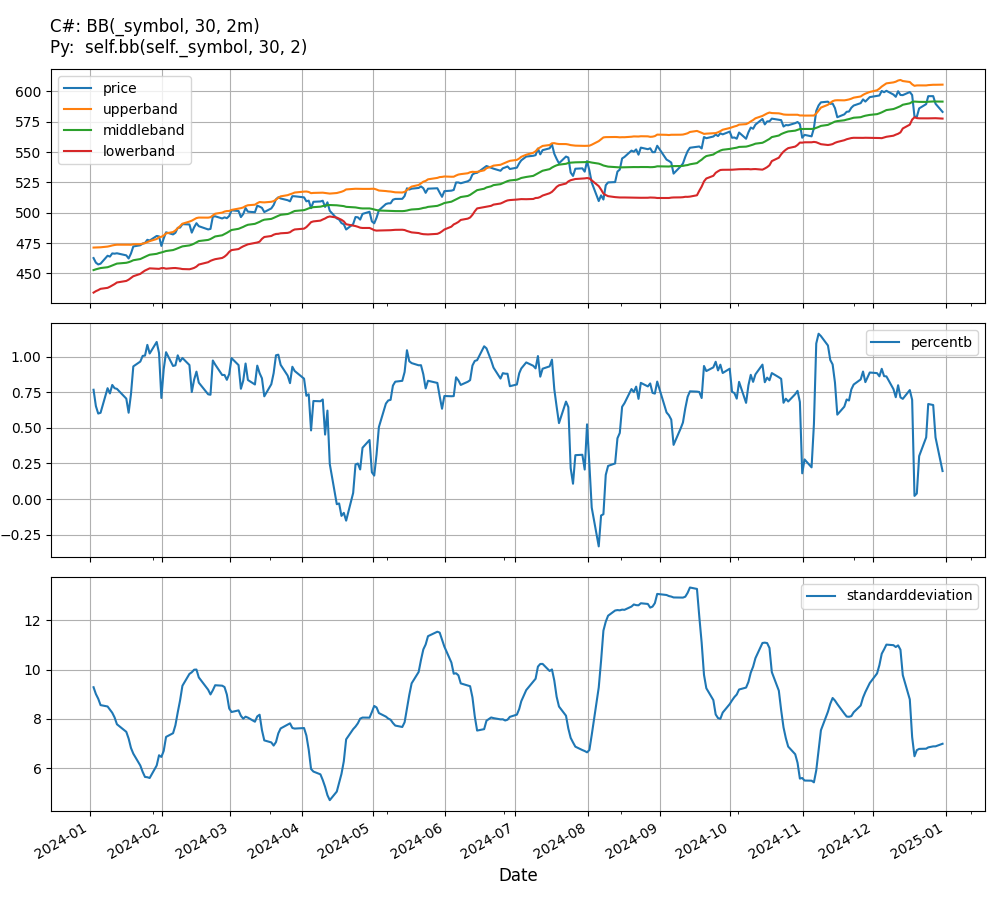# Supported Indicators

## Bollinger Bands

### Introduction

This indicator creates a moving average (middle band) with an upper band and lower band fixed at k standard deviations above and below the moving average.

To view the implementation of this indicator, see the LEAN GitHub repository.

### Using BB Indicator

To create an automatic indicators for BollingerBands, call the BB helper method from the QCAlgorithm class. The BB method creates a BollingerBands object, hooks it up for automatic updates, and returns it so you can used it in your algorithm. In most cases, you should call the helper method in the Initialize method.

public class BollingerBandsAlgorithm : QCAlgorithm
{
private Symbol _symbol;
private BollingerBands _bb;

public override void Initialize()
{
_bb = BB(_symbol, 30, 2);
}

public override void OnData(Slice data)
{
{
// The current value of _bb is represented by itself (_bb)
// or _bb.Current.Value
Plot("BollingerBands", "bb", _bb);
// Plot all properties of bb
Plot("BollingerBands", "standarddeviation", _bb.StandardDeviation);
Plot("BollingerBands", "middleband", _bb.MiddleBand);
Plot("BollingerBands", "upperband", _bb.UpperBand);
Plot("BollingerBands", "lowerband", _bb.LowerBand);
Plot("BollingerBands", "bandwidth", _bb.BandWidth);
Plot("BollingerBands", "percentb", _bb.PercentB);
Plot("BollingerBands", "price", _bb.Price);
}
}
}
class BollingerBandsAlgorithm(QCAlgorithm):
def Initialize(self) -> None:
self.bb = self.BB(self.symbol, 30, 2)

def OnData(self, slice: Slice) -> None:
# The current value of self.bb is represented by self.bb.Current.Value
self.Plot("BollingerBands", "bb", self.bb.Current.Value)
# Plot all attributes of self.bb
self.Plot("BollingerBands", "standarddeviation", self.bb.StandardDeviation.Current.Value)
self.Plot("BollingerBands", "middleband", self.bb.MiddleBand.Current.Value)
self.Plot("BollingerBands", "upperband", self.bb.UpperBand.Current.Value)
self.Plot("BollingerBands", "lowerband", self.bb.LowerBand.Current.Value)
self.Plot("BollingerBands", "bandwidth", self.bb.BandWidth.Current.Value)
self.Plot("BollingerBands", "percentb", self.bb.PercentB.Current.Value)
self.Plot("BollingerBands", "price", self.bb.Price.Current.Value)


The following reference table describes the BB method:

### BB()1/1

            BollingerBands QuantConnect.Algorithm.QCAlgorithm.BB (
Symbol                           symbol,
Int32                            period,
Decimal                          k,
*MovingAverageType               movingAverageType,
*Nullable<Resolution>      resolution,
*Func<IBaseData, Decimal>  selector
)


Creates a new BollingerBands indicator which will compute the MiddleBand, UpperBand, LowerBand, and StandardDeviation.

If you don't provide a resolution, it defaults to the security resolution. If you provide a resolution, it must be greater than or equal to the resolution of the security. For instance, if you subscribe to hourly data for a security, you should update its indicator with data that spans 1 hour or longer.

The following table describes the MovingAverageType enumeration members:

You can manually create a BollingerBands indicator, so it doesn't automatically update. Manual indicators let you update their values with any data you choose.

Updating your indicator manually enables you to control when the indicator is updated and what data you use to update it. To manually update the indicator, call the Update method with time/number pair, or an IndicatorDataPoint. The indicator will only be ready after you prime it with enough data.

public class BollingerBandsAlgorithm : QCAlgorithm
{
private Symbol _symbol;
private BollingerBands _bb;

public override void Initialize()
{
_bb = new BollingerBands(30, 2);
}

public override void OnData(Slice data)
{
if (data.Bars.TryGeValue(_symbol, out var bar))
{
_bb.Update(bar.EndTime, bar.Close);
}

{
// The current value of _bb is represented by itself (_bb)
// or _bb.Current.Value
Plot("BollingerBands", "bb", _bb);
// Plot all properties of bb
Plot("BollingerBands", "standarddeviation", _bb.StandardDeviation);
Plot("BollingerBands", "middleband", _bb.MiddleBand);
Plot("BollingerBands", "upperband", _bb.UpperBand);
Plot("BollingerBands", "lowerband", _bb.LowerBand);
Plot("BollingerBands", "bandwidth", _bb.BandWidth);
Plot("BollingerBands", "percentb", _bb.PercentB);
Plot("BollingerBands", "price", _bb.Price);
}
}
}
class BollingerBandsAlgorithm(QCAlgorithm):
def Initialize(self) -> None:
self.bb = BollingerBands(30, 2)

def OnData(self, slice: Slice) -> None:
bar = slice.Bars.get(self.symbol)
if bar:
self.bb.Update(bar.EndTime, bar.Close)

# The current value of self.bb is represented by self.bb.Current.Value
self.Plot("BollingerBands", "bb", self.bb.Current.Value)
# Plot all attributes of self.bb
self.Plot("BollingerBands", "standarddeviation", self.bb.StandardDeviation.Current.Value)
self.Plot("BollingerBands", "middleband", self.bb.MiddleBand.Current.Value)
self.Plot("BollingerBands", "upperband", self.bb.UpperBand.Current.Value)
self.Plot("BollingerBands", "lowerband", self.bb.LowerBand.Current.Value)
self.Plot("BollingerBands", "bandwidth", self.bb.BandWidth.Current.Value)
self.Plot("BollingerBands", "percentb", self.bb.PercentB.Current.Value)
self.Plot("BollingerBands", "price", self.bb.Price.Current.Value)


To register a manual indicator for automatic updates with the security data, call the RegisterIndicator method.

public class BollingerBandsAlgorithm : QCAlgorithm
{
private Symbol _symbol;
private BollingerBands _bb;

public override void Initialize()
{
_bb = new BollingerBands(30, 2);
RegisterIndicator(_symbol, _bb, Resolution.Daily);
}

public override void OnData(Slice data)
{
{
// The current value of _bb is represented by itself (_bb)
// or _bb.Current.Value
Plot("BollingerBands", "bb", _bb);
// Plot all properties of bb
Plot("BollingerBands", "standarddeviation", _bb.StandardDeviation);
Plot("BollingerBands", "middleband", _bb.MiddleBand);
Plot("BollingerBands", "upperband", _bb.UpperBand);
Plot("BollingerBands", "lowerband", _bb.LowerBand);
Plot("BollingerBands", "bandwidth", _bb.BandWidth);
Plot("BollingerBands", "percentb", _bb.PercentB);
Plot("BollingerBands", "price", _bb.Price);
}
}
}
class BollingerBandsAlgorithm(QCAlgorithm):
def Initialize(self) -> None:
self.bb = BollingerBands(30, 2)
self.RegisterIndicator(self.symbol, self.bb, Resolution.Daily)

def OnData(self, slice: Slice) -> None:
# The current value of self.bb is represented by self.bb.Current.Value
self.Plot("BollingerBands", "bb", self.bb.Current.Value)
# Plot all attributes of self.bb
self.Plot("BollingerBands", "standarddeviation", self.bb.StandardDeviation.Current.Value)
self.Plot("BollingerBands", "middleband", self.bb.MiddleBand.Current.Value)
self.Plot("BollingerBands", "upperband", self.bb.UpperBand.Current.Value)
self.Plot("BollingerBands", "lowerband", self.bb.LowerBand.Current.Value)
self.Plot("BollingerBands", "bandwidth", self.bb.BandWidth.Current.Value)
self.Plot("BollingerBands", "percentb", self.bb.PercentB.Current.Value)
self.Plot("BollingerBands", "price", self.bb.Price.Current.Value)


The following reference table describes the BollingerBands constructor:

### BollingerBands()1/2

            BollingerBands QuantConnect.Indicators.BollingerBands (
int                 period,
decimal             k,
*MovingAverageType  movingAverageType
)


Initializes a new instance of the BollingerBands class.

### BollingerBands()2/2

            BollingerBands QuantConnect.Indicators.BollingerBands (
string              name,
int                 period,
decimal             k,
*MovingAverageType  movingAverageType
)


Initializes a new instance of the BollingerBands class.

### Visualization

The following image shows plot values of selected properties of BollingerBands using the plotly library.You can also see our Videos. You can also get in touch with us via Discord.